# 如何用Python和深度神经网络锁定即将流失的客户？

+关注继续查看

Python深度学习简明实战教程来了。别犹豫了，赶紧从零开始，搭建你自己的第一个深度学习模型吧！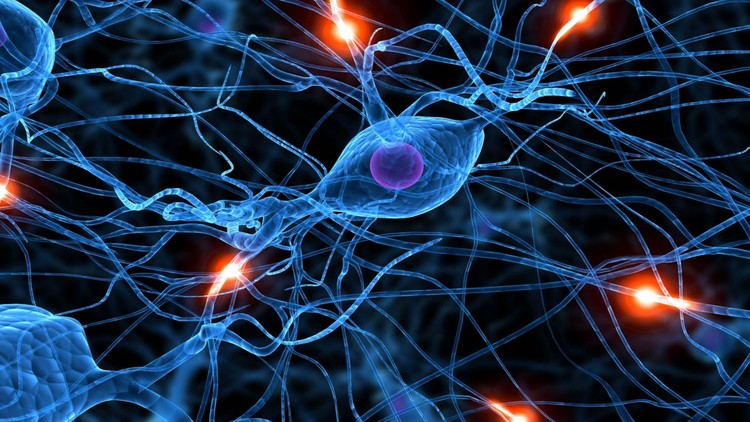# 烦恼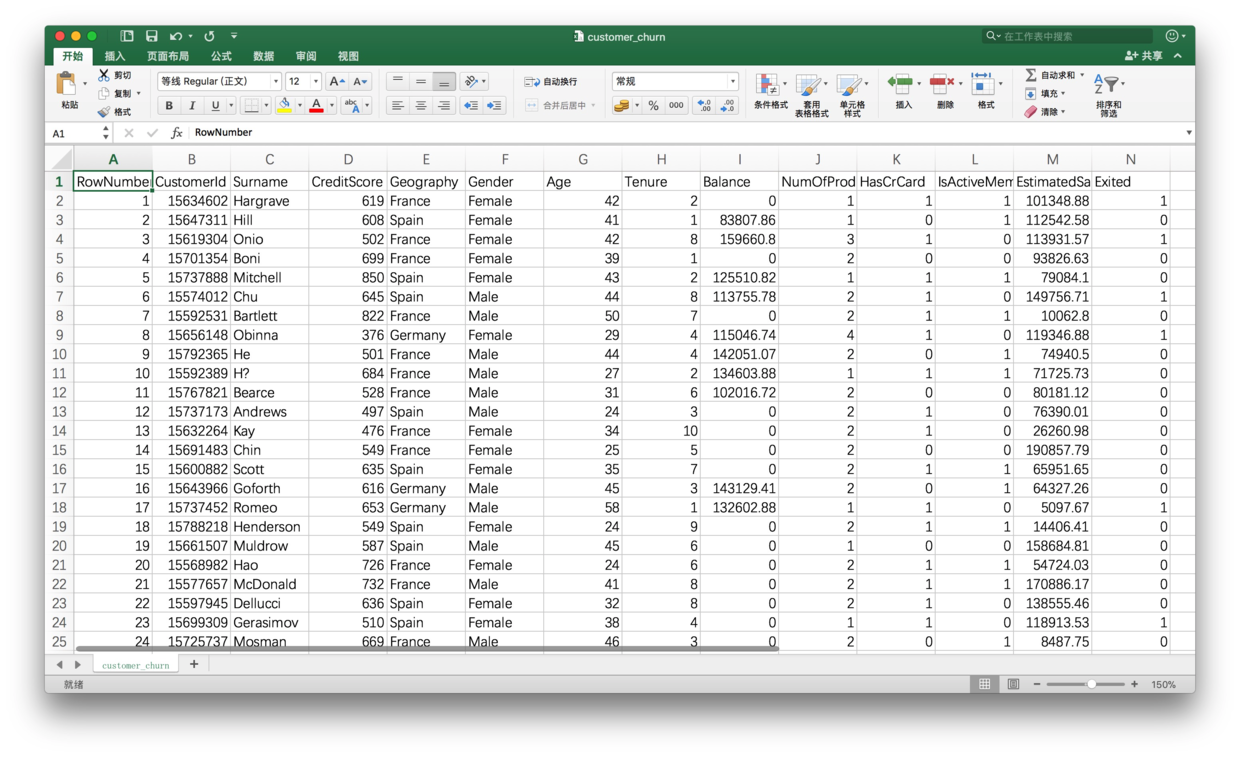# 环境（注：样例数据来自于匿名化处理后的真实数据集，下载自superdatascience官网。）

jupyter notebook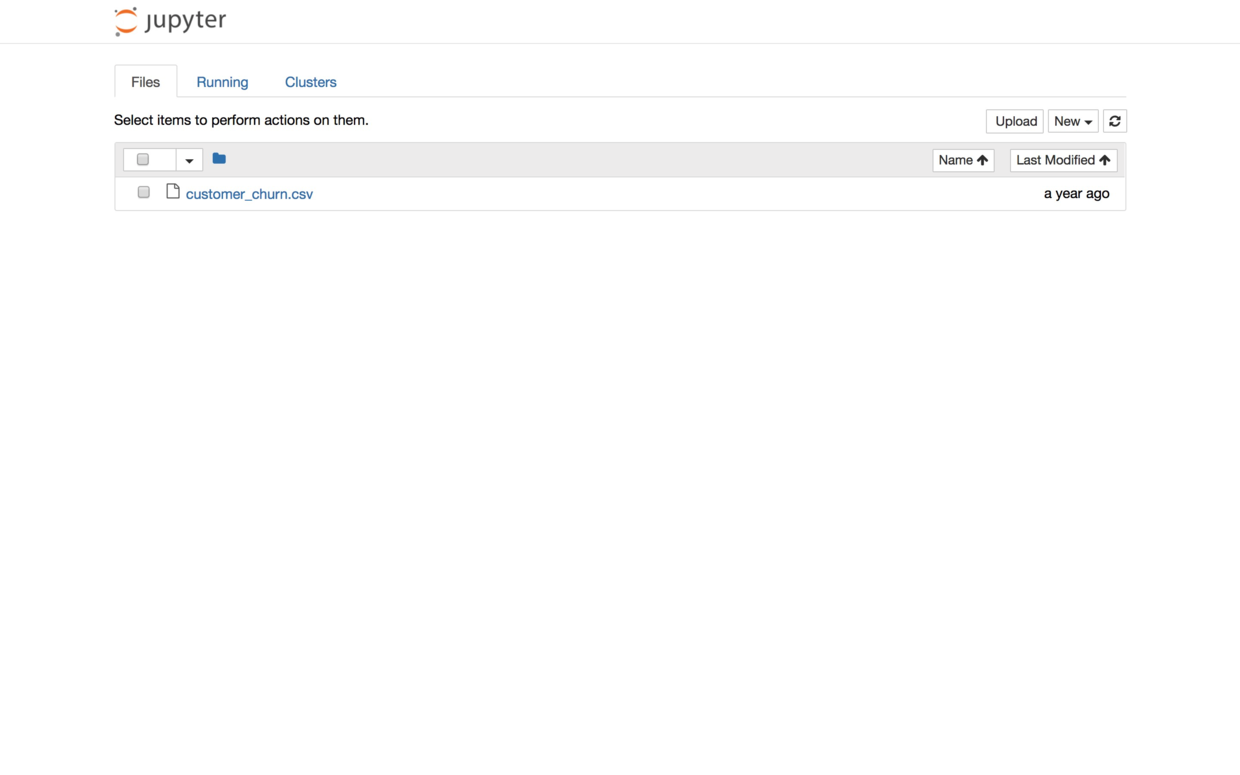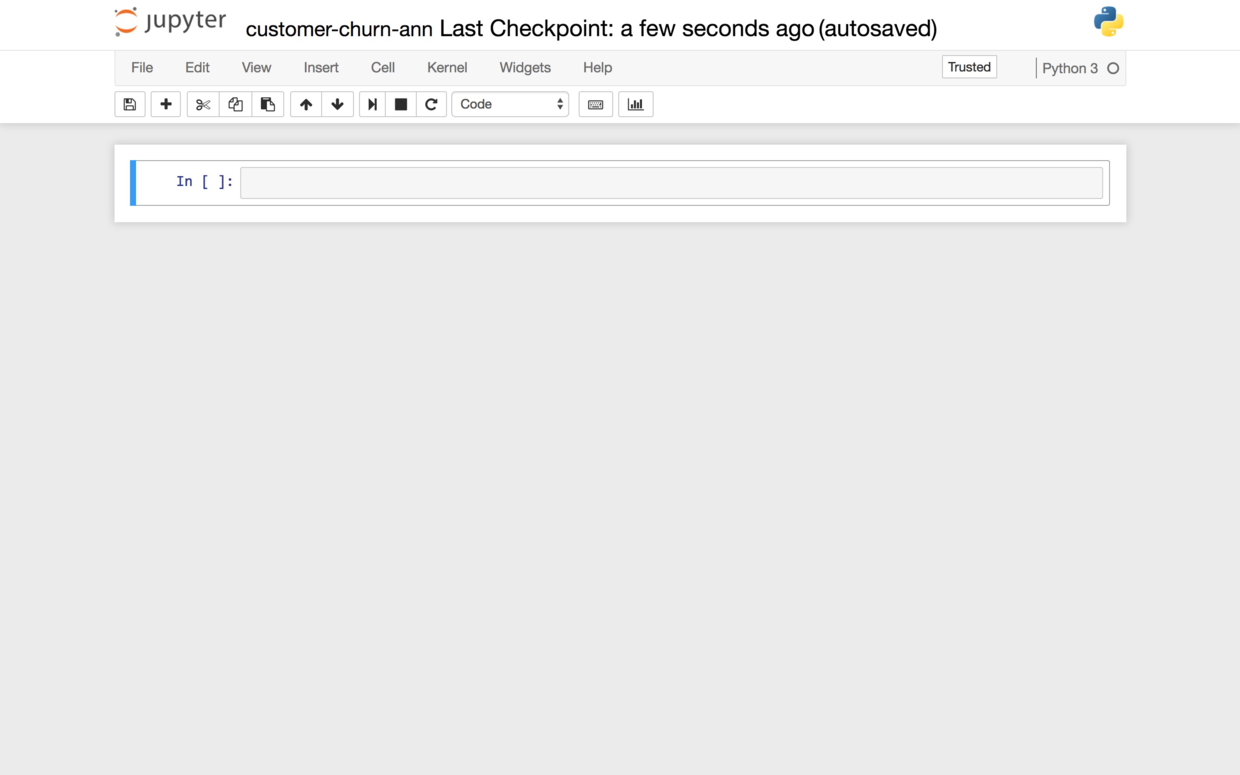# 清理

import numpy as np
import pandas as pd


customer_churn.csv里读入数据：

df = pd.read_csv('customer_churn.csv')


df.head()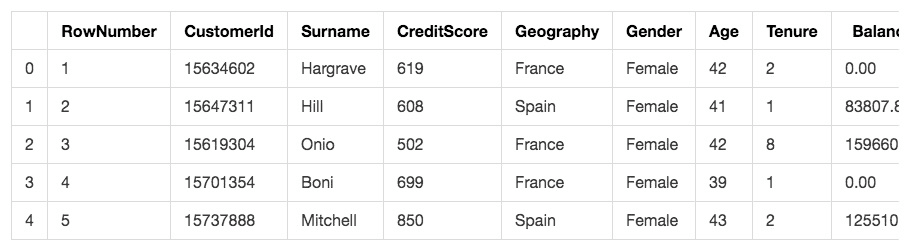• RowNumber：行号，这个肯定没用，删除
• CustomerID：用户编号，这个是顺序发放的，删除
• Surname：用户姓名，对流失没有影响，删除
• CreditScore：信用分数，这个很重要，保留
• Geography：用户所在国家/地区，这个有影响，保留
• Gender：用户性别，可能有影响，保留
• Age：年龄，影响很大，年轻人更容易切换银行，保留
• Tenure：当了本银行多少年用户，很重要，保留
• Balance：存贷款情况，很重要，保留
• NumOfProducts：使用产品数量，很重要，保留
• HasCrCard：是否有本行信用卡，很重要，保留
• IsActiveMember：是否活跃用户，很重要，保留
• EstimatedSalary：估计收入，很重要，保留
• Exited：是否已流失，这将作为我们的标签数据

X = df.loc[:,['CreditScore', 'Geography', 'Gender', 'Age', 'Tenure', 'Balance', 'NumOfProducts', 'HasCrCard', 'IsActiveMember', 'EstimatedSalary']]


X.head()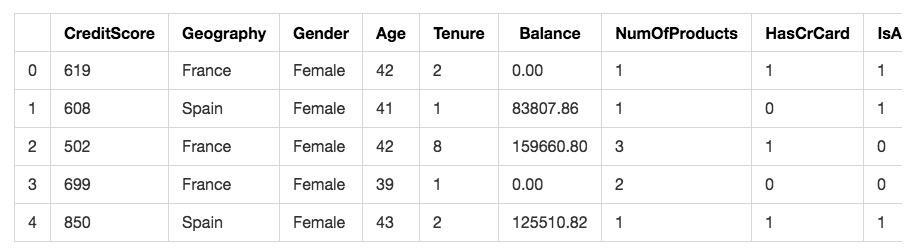2017-11-19_19-2-2_snapshots-01.jpg

y = df.Exited

y.head()

0    1
1    0
2    1
3    0
4    0
Name: Exited, dtype: int64


X.head()2017-11-19_19-2-2_snapshots-01.jpg

from sklearn.preprocessing import LabelEncoder, OneHotEncoder
labelencoder1 = LabelEncoder()
X.Geography= labelencoder1.fit_transform(X.Geography)
labelencoder2 = LabelEncoder()
X.Gender = labelencoder2.fit_transform(X.Gender)


X.head()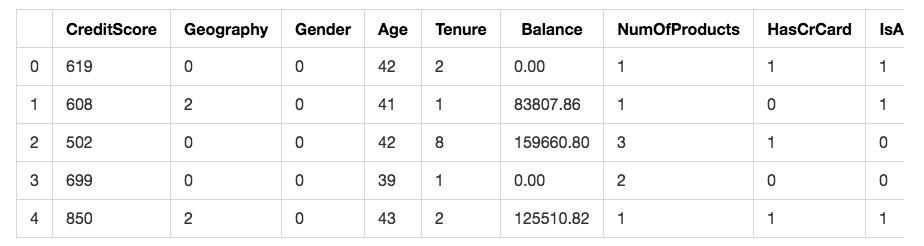2017-11-19_19-2-11_snapshots-03.jpg

onehotencoder = OneHotEncoder(categorical_features = )
X = onehotencoder.fit_transform(X).toarray()


X

array([  1.00000000e+00,   0.00000000e+00,   0.00000000e+00,
6.19000000e+02,   0.00000000e+00,   4.20000000e+01,
2.00000000e+00,   0.00000000e+00,   1.00000000e+00,
1.00000000e+00,   1.00000000e+00,   1.01348880e+05])


X = np.delete(X, , 1)


X

array([  0.00000000e+00,   0.00000000e+00,   6.19000000e+02,
0.00000000e+00,   4.20000000e+01,   2.00000000e+00,
0.00000000e+00,   1.00000000e+00,   1.00000000e+00,
1.00000000e+00,   1.01348880e+05])


y.head()

0    1
1    0
2    1
3    0
4    0
Name: Exited, dtype: int64


y = y[:, np.newaxis]
y

array([,
,
,
...,
,
,
])


onehotencoder = OneHotEncoder()
y = onehotencoder.fit_transform(y).toarray()


y

array([[ 0.,  1.],
[ 1.,  0.],
[ 0.,  1.],
...,
[ 0.,  1.],
[ 0.,  1.],
[ 1.,  0.]])


from sklearn.model_selection import train_test_split
X_train, X_test, y_train, y_test = train_test_split(X, y, test_size = 0.2, random_state = 0)


len(X_train)

8000


len(X_test)

2000


from sklearn.preprocessing import StandardScaler
sc = StandardScaler()
X_train = sc.fit_transform(X_train)
X_test = sc.transform(X_test)


X_train

array([[-0.5698444 ,  1.74309049,  0.16958176, ...,  0.64259497,
-1.03227043,  1.10643166],
[ 1.75486502, -0.57369368, -2.30455945, ...,  0.64259497,
0.9687384 , -0.74866447],
[-0.5698444 , -0.57369368, -1.19119591, ...,  0.64259497,
-1.03227043,  1.48533467],
...,
[-0.5698444 , -0.57369368,  0.9015152 , ...,  0.64259497,
-1.03227043,  1.41231994],
[-0.5698444 ,  1.74309049, -0.62420521, ...,  0.64259497,
0.9687384 ,  0.84432121],
[ 1.75486502, -0.57369368, -0.28401079, ...,  0.64259497,
-1.03227043,  0.32472465]])


# 决策树

from sklearn import tree
clf = tree.DecisionTreeClassifier()
clf = clf.fit(X_train, y_train)


y_pred = clf.predict(X_test)


y_pred

array([[ 1.,  0.],
[ 0.,  1.],
[ 1.,  0.],
...,
[ 1.,  0.],
[ 1.,  0.],
[ 0.,  1.]])


from sklearn.metrics import classification_report
print(classification_report(y_test, y_pred))

             precision    recall  f1-score   support

          0       0.89      0.86      0.87      1595
1       0.51      0.58      0.54       405

avg / total       0.81      0.80      0.81      2000


# 深度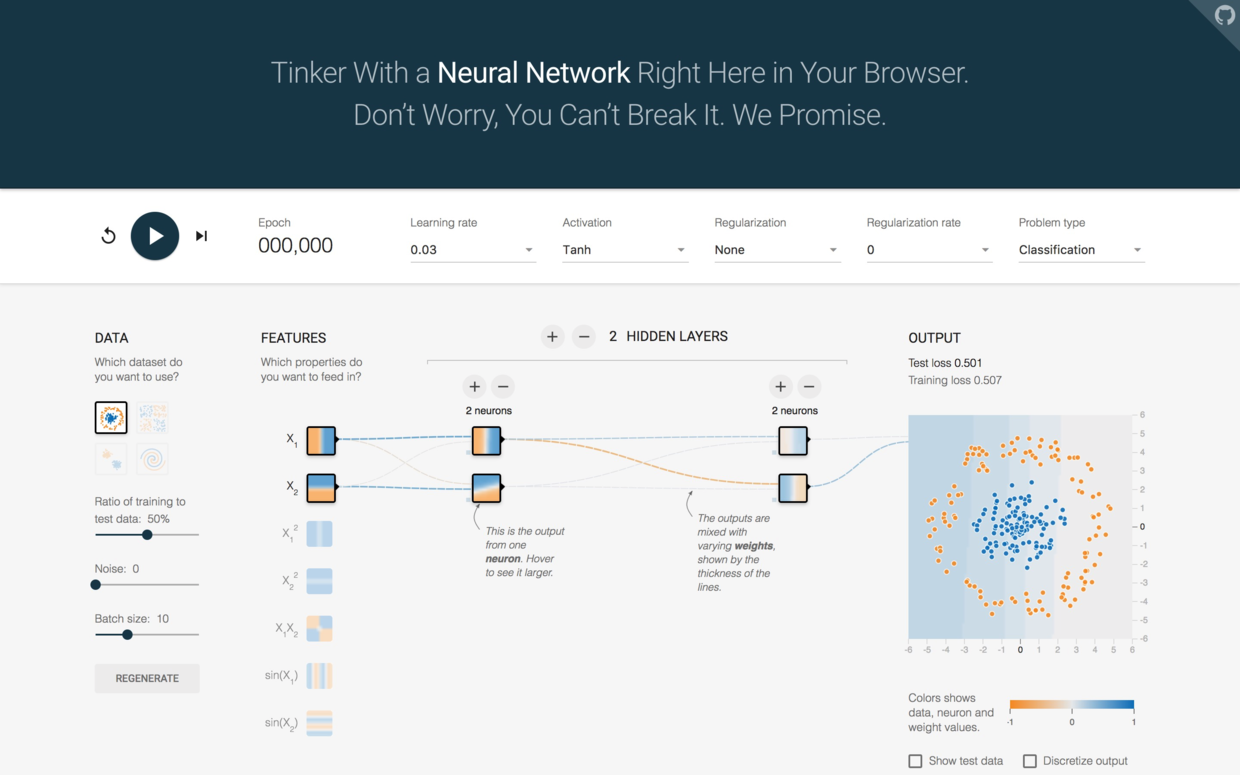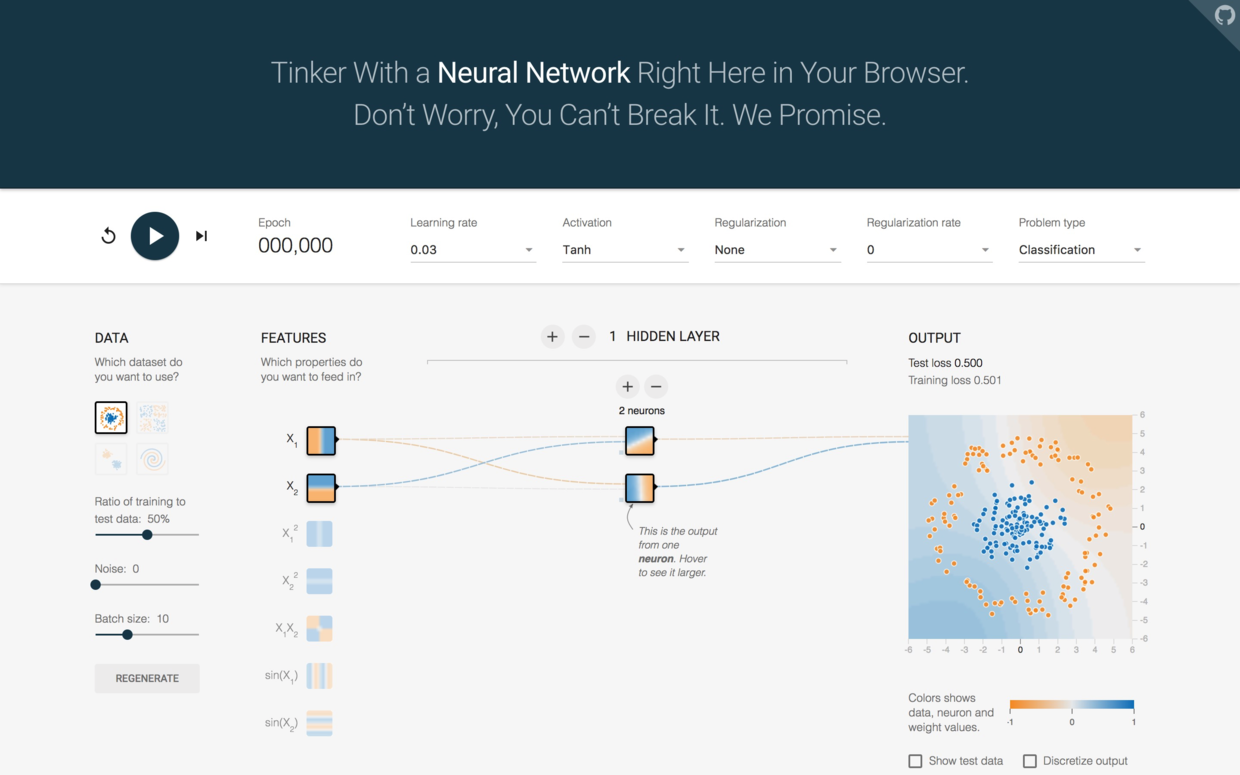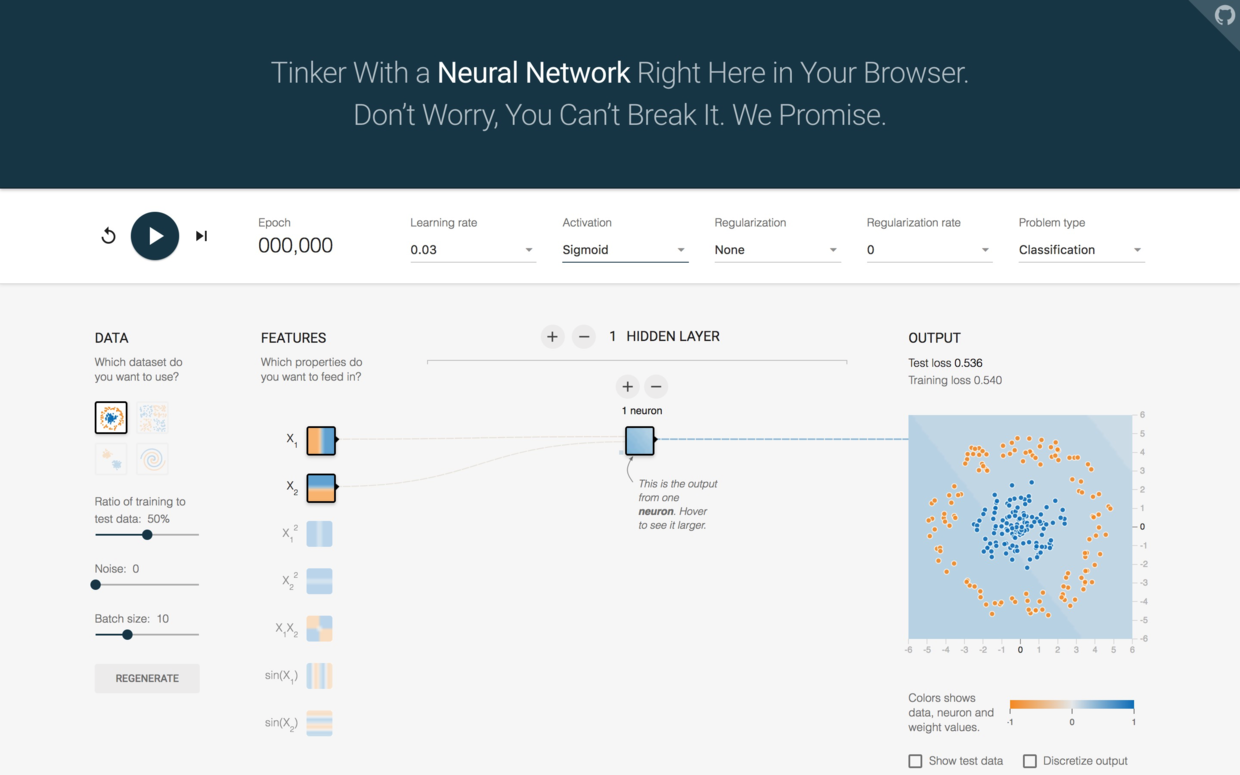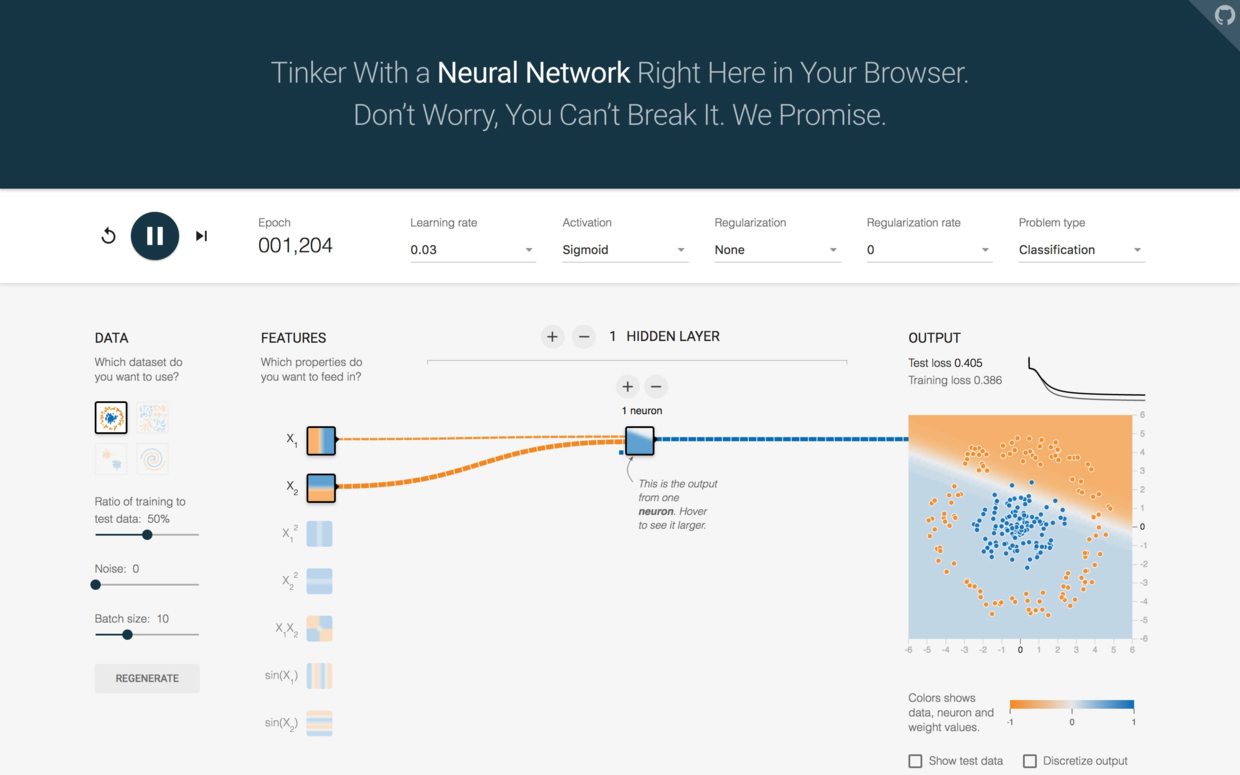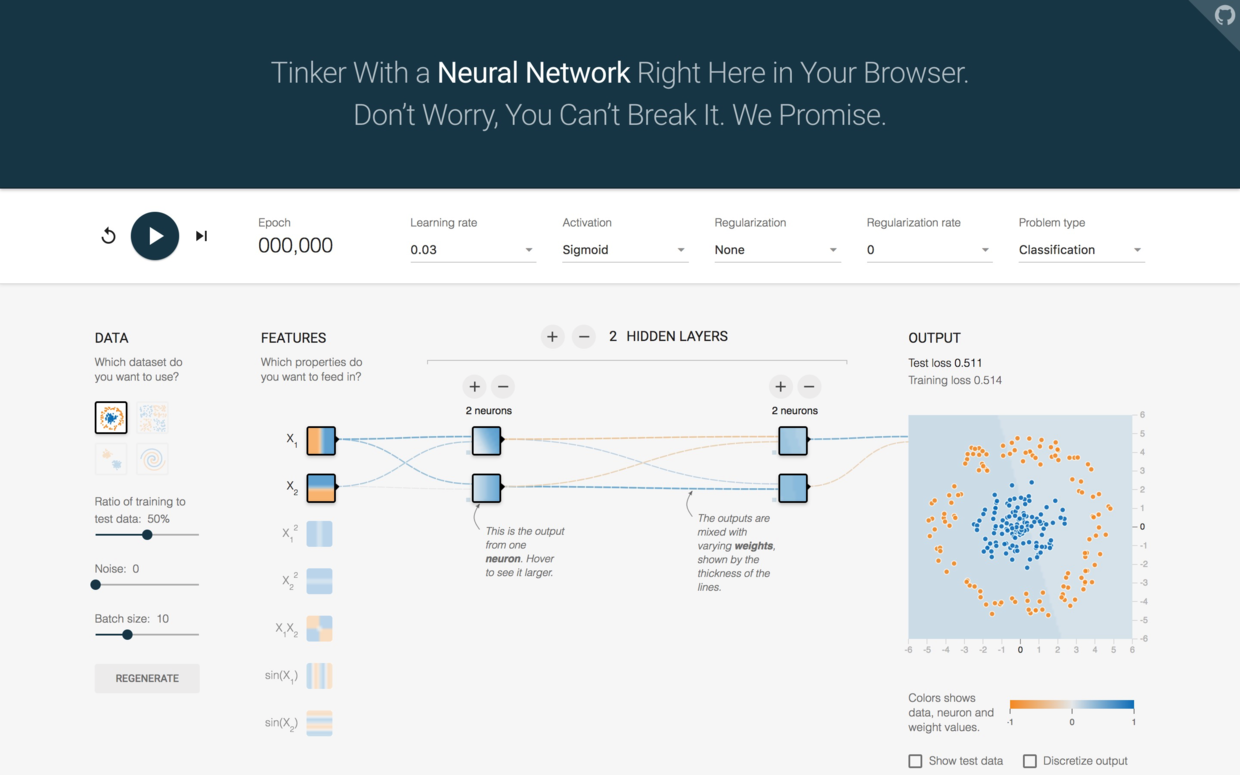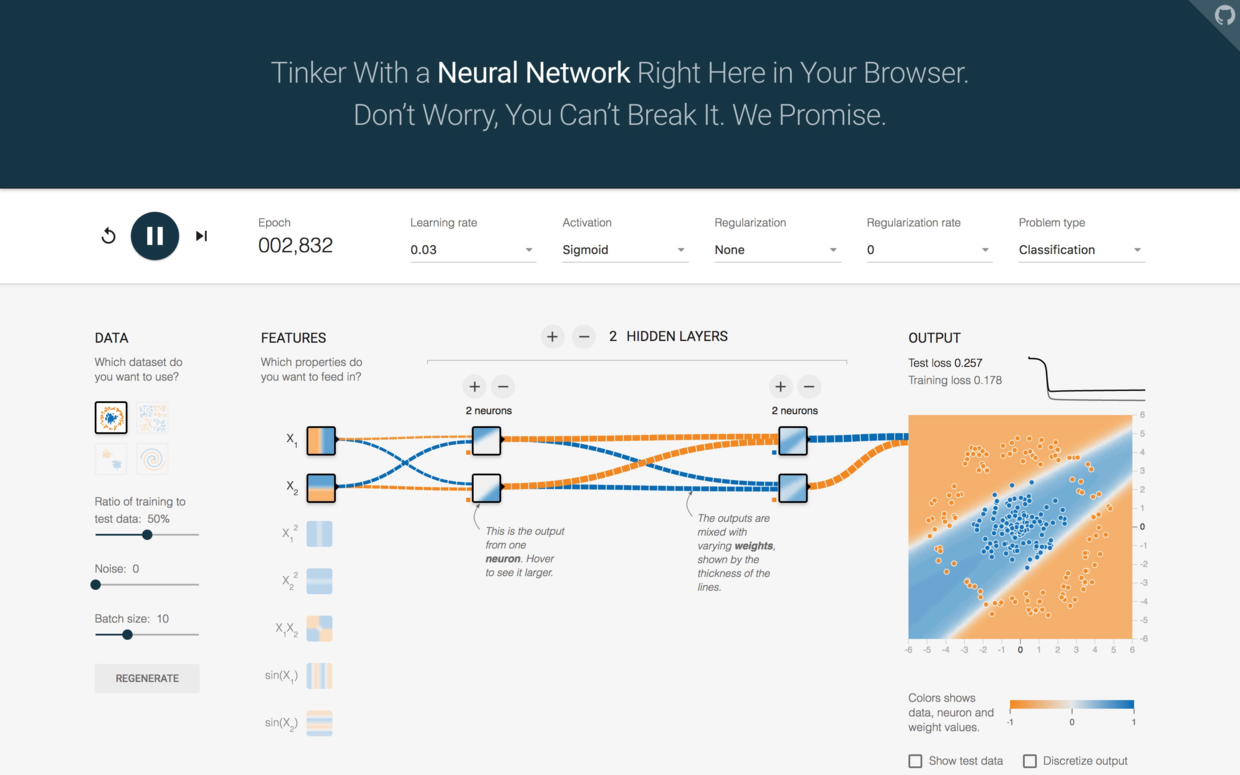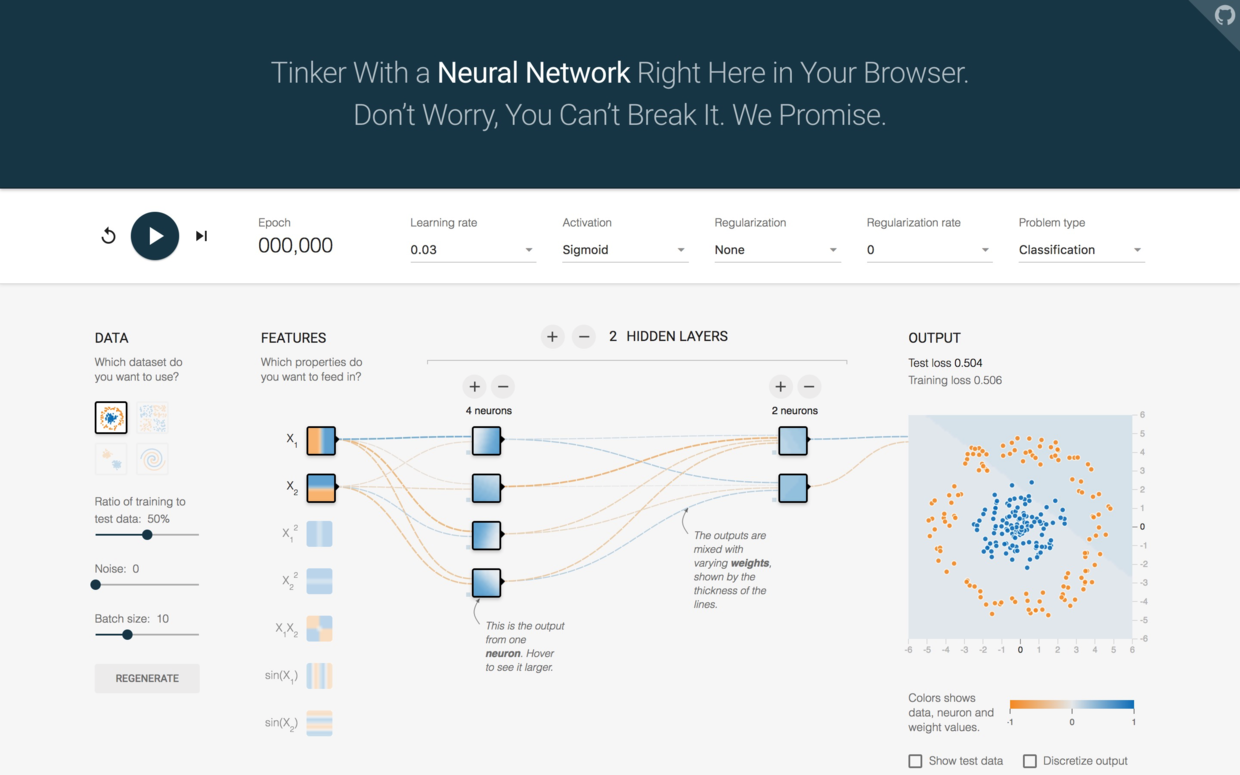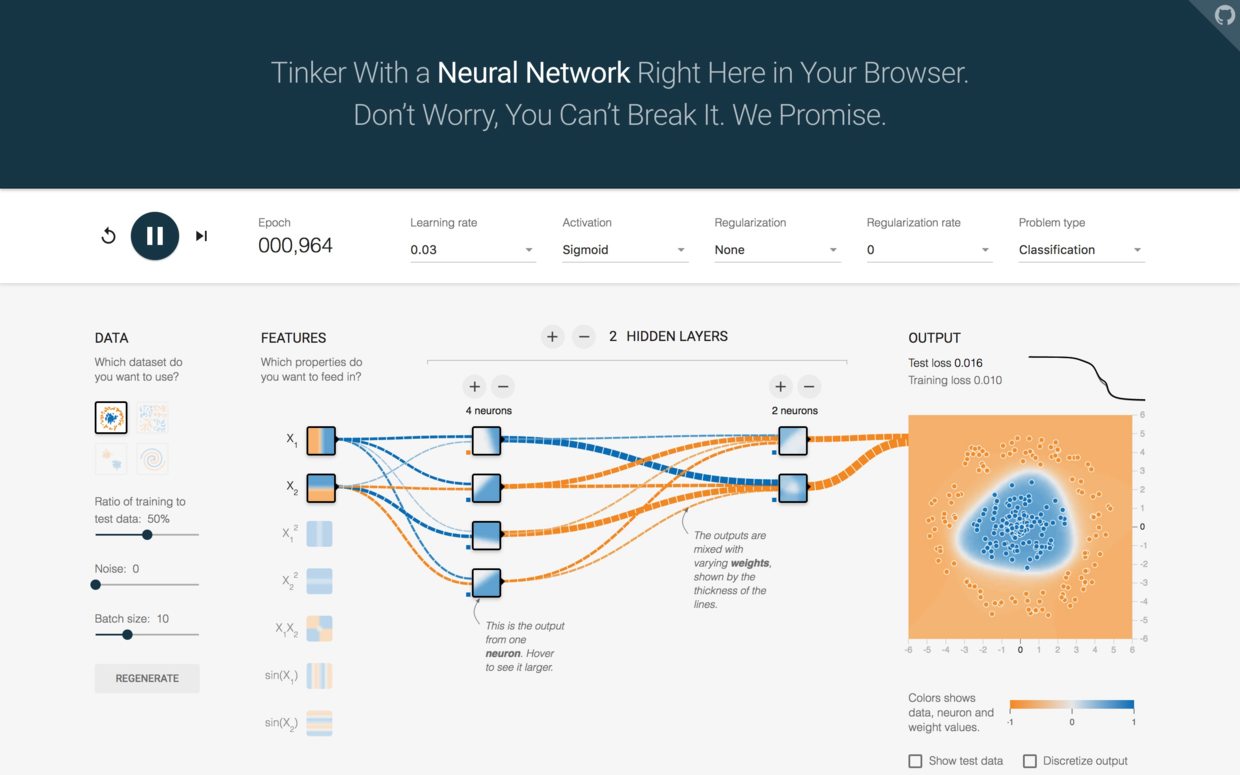# 实战

pip install tensorflow
pip install tflearn


import tflearn


net = tflearn.input_data(shape=[None, 11])


shape的第一项，None，指的是我们要输入的特征矩阵行数。因为我们现在是搭建模型，后面特征矩阵有可能一次输入，有可能分成组块输入，长度可大可小，无法事先确定。所以这里填None。tflearn会在我们实际执行训练的时候，自己读入特征矩阵的尺寸，来处理这个数值。

net = tflearn.fully_connected(net, 6, activation='relu')
net = tflearn.fully_connected(net, 6, activation='relu')
net = tflearn.fully_connected(net, 6, activation='relu')


activation刚才在深度学习游乐场里面我们遇到过，代表激活函数。如果没有它，所有的输入输出都是线性关系。

Relu函数是激活函数的一种。它大概长这个样子。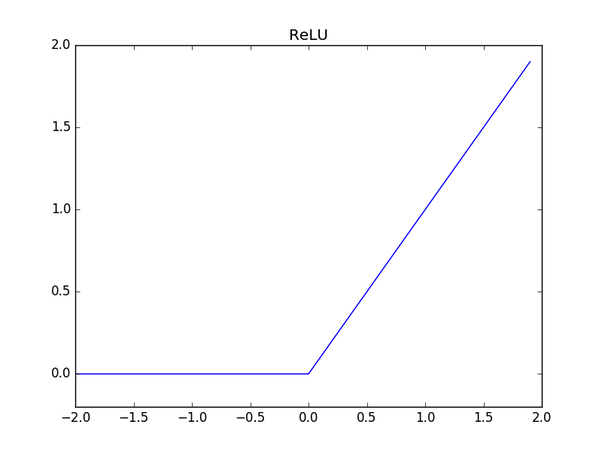net = tflearn.fully_connected(net, 2, activation='softmax')
net = tflearn.regression(net)


model = tflearn.DNN(net)


model.fit(X_train, y_train, n_epoch=30, batch_size=32, show_metric=True)


• n_epoch：数据训练几个轮次。
• batch_size：每一次输入给模型的数据行数。
• show_metric：训练过程中要不要打印结果。

Training Step: 7499  | total loss: �[1m�[32m0.39757�[0m�[0m | time: 0.656s
| Adam | epoch: 030 | loss: 0.39757 - acc: 0.8493 -- iter: 7968/8000
Training Step: 7500  | total loss: �[1m�[32m0.40385�[0m�[0m | time: 0.659s
| Adam | epoch: 030 | loss: 0.40385 - acc: 0.8487 -- iter: 8000/8000
--


tensorboard --logdir=/tmp/tflearn_logs/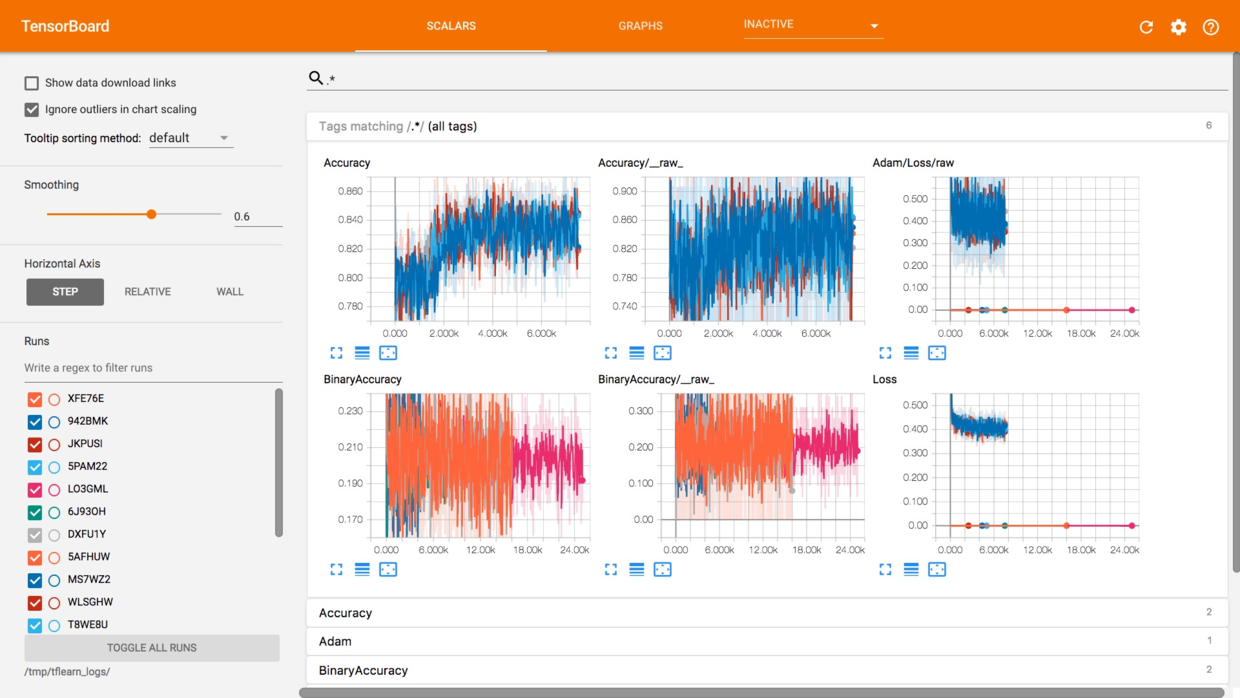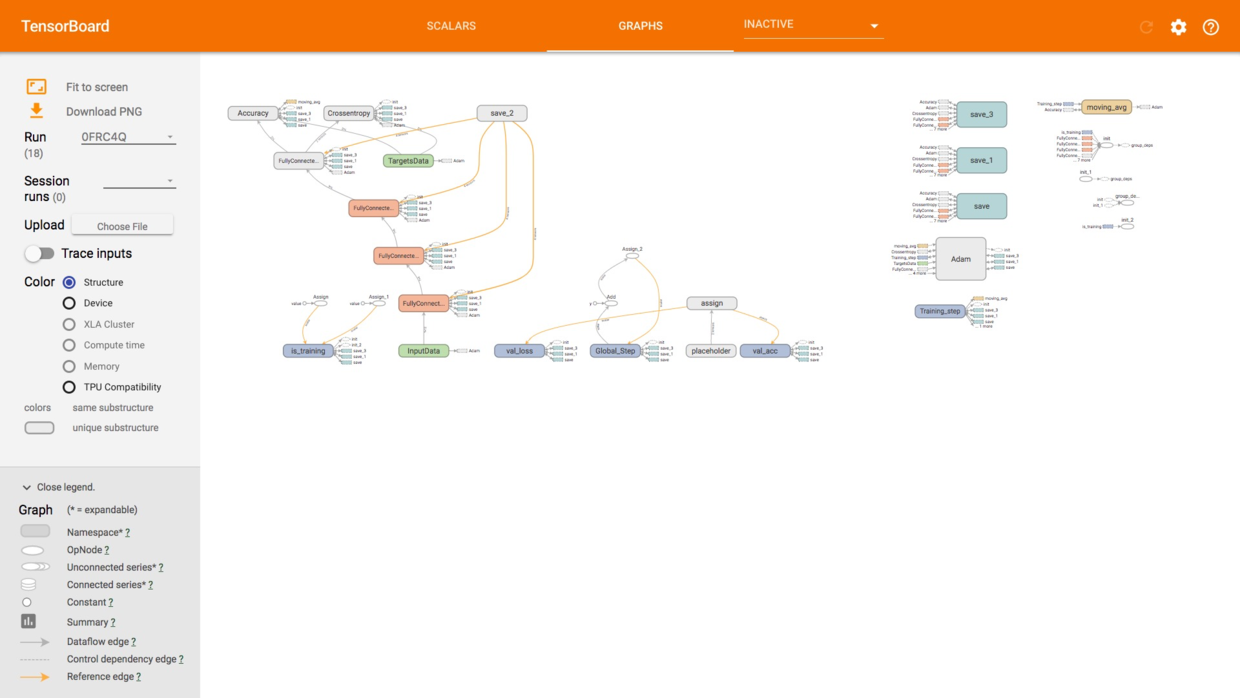# 评估

X_test

array([ 1.75486502, -0.57369368, -0.55204276, -1.09168714, -0.36890377,
1.04473698,  0.8793029 , -0.92159124,  0.64259497,  0.9687384 ,
1.61085707])


y_pred = model.predict(X_test)


y_pred

array([ 0.70956731,  0.29043278], dtype=float32)


y_test

array([ 1.,  0.])


score = model.evaluate(X_test, y_test)
print('Test accuarcy: %0.4f%%' % (score * 100))

Test accuarcy: 84.1500%


# 学习资源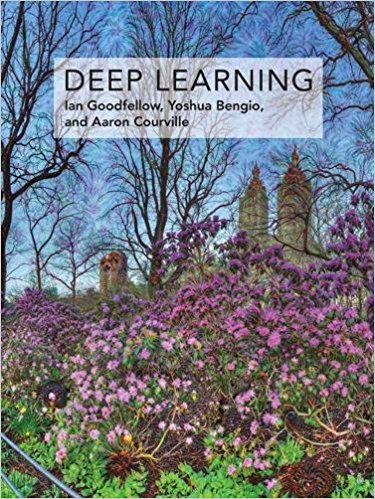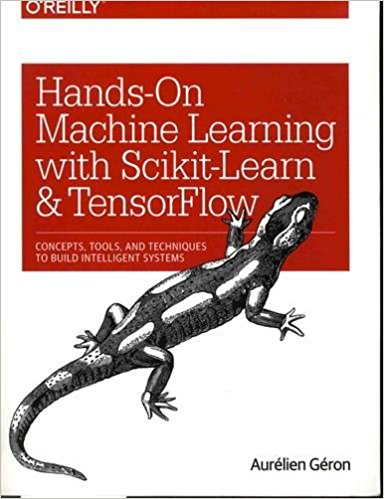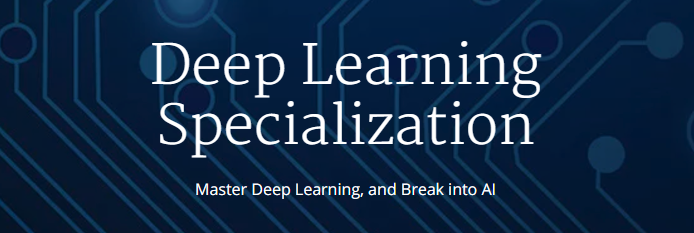# 讨论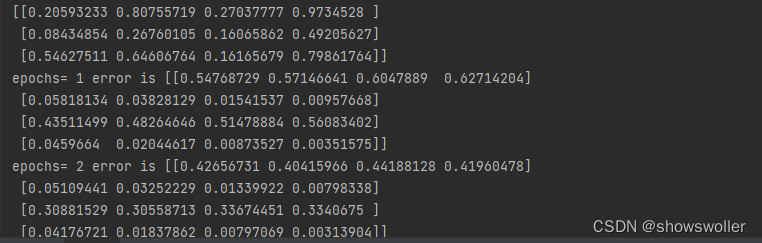python神经网络之实现双层感知器和神经网络感知器算法将鸢尾分类
python神经网络之实现双层感知器和神经网络感知器算法将鸢尾分类
0 0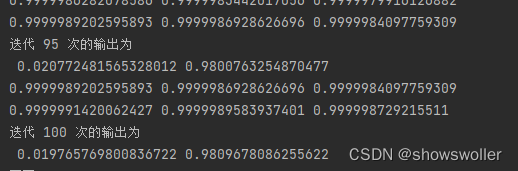python实现BP神经网络进行预测和误差分析（附源代码）
python实现BP神经网络进行预测和误差分析（附源代码）
0 0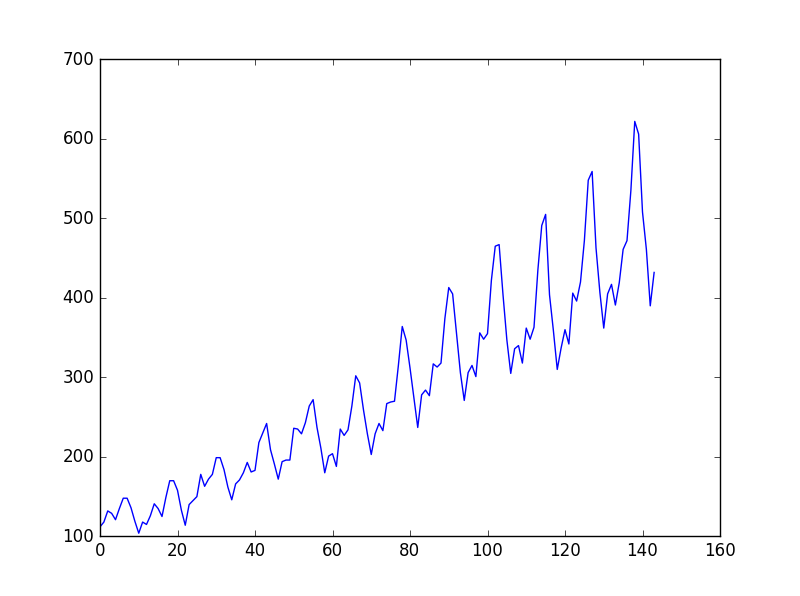Python中LSTM回归神经网络的时间序列预测
Python中LSTM回归神经网络的时间序列预测
0 00 0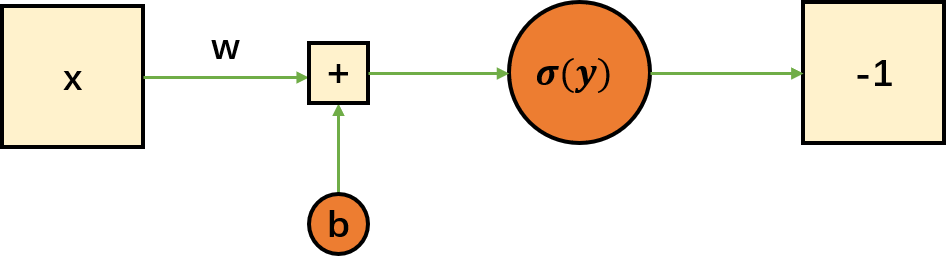Python快速构建神经网络

0 0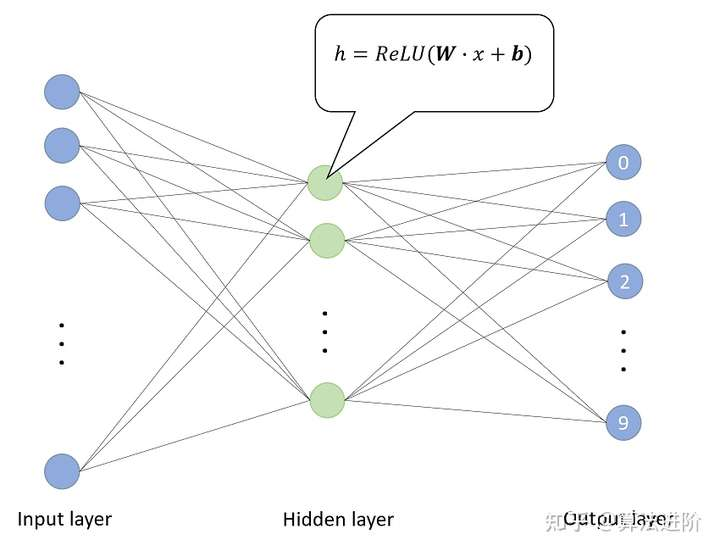0 0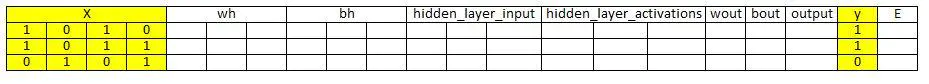0 0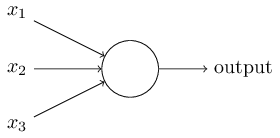0 00 0

【科技少年】Python绘画编程第一课252520

【科技少年】Python基础语法128524422101

【视觉与图像】Python+OpenCV教程入门篇-1月16日更新7916

【视觉与图像】Python+OpenCV教程基础篇861415654

Python 脚本速查手册